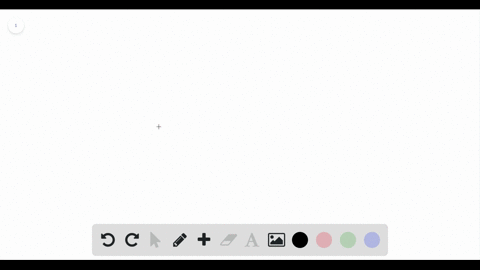Enroll in one of our FREE online STEM bootcamps. Join today and start acing your classes!View Bootcamps## Discussion

You must be signed in to discuss.

## Video Transcript

question 20. They give us a triangle and again they draw it where the base is here. The height is here, okay. And the high should be perpendicular to the base. They ask us to express the issue of the height to the base in simple swarm where the height, the height is 40 millimeters and the base is 0.2 meters. Okay, So something you need to know before going into this is that there are 1000 millimetres zeros Funny. Okay, 1000 millimetres in one meter. Okay, so you need to have the same unit. So one thing you can do to change these meters here and two millimeters is moved three places to the right. That's how you convert meters two millimeters. So if you moved three places to the right, you'll get 123 so you'll get 200 millimeters Now it wants the ratio off 40 2 200 which is 1 to 5